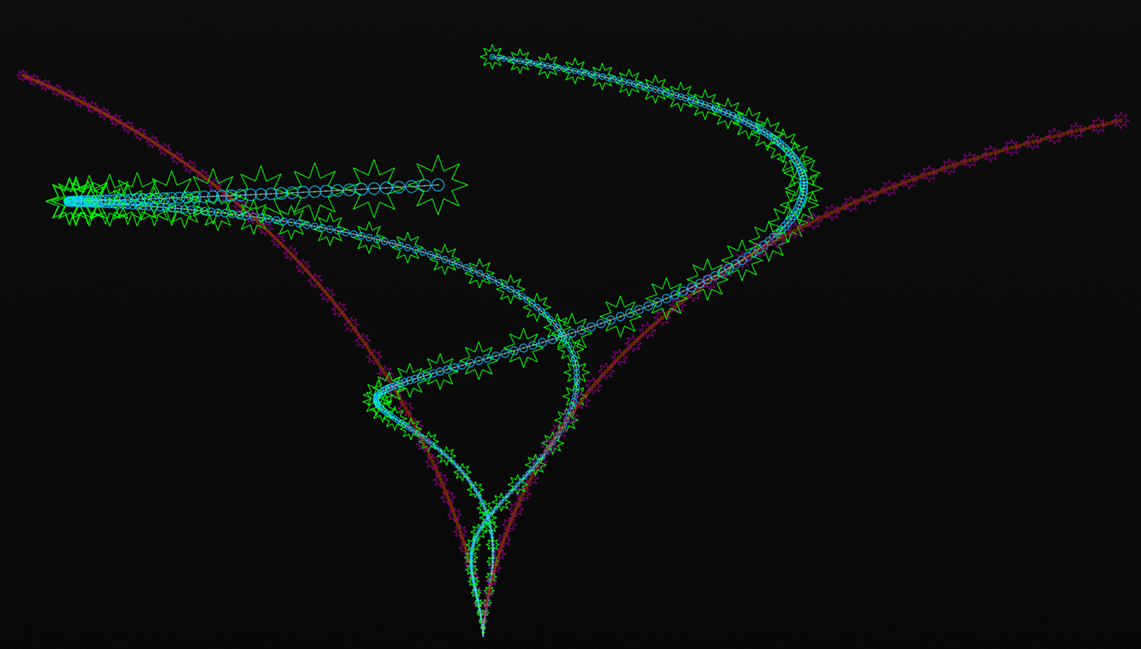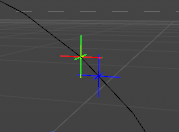# SOLVEDFrom Line Object to Spline

(edited by Manuel : I splited this thread from this one)

@m_magalhaes said in Post Deformer Spline:

SplineToLineIndex

Hi Manuel,

I am not the one who asked the original question, but your find of the `SplineToLineIndex()` method in `SplineHelp` is a good one!

I must have look at `SplineHelp`'s API over a dozen times and never noticed it was there. I thought one would have to traverse all of the `LineObject`'s points and correlate them with points on its parent `SplineObject` based on position, in order to come up with the mapping from `SplineObject` point index to `LineObject` point index. So, this feature of `SplineHelp` is really valuable, since it offers this very mapping as part of the core Python API.

I guess this also implies that there is always a 1:1 correspondence between a point of a SplineObject (which represents one or more B-Splines via formulas) and a point of its underlying LineObject which represents a bunch of line segments connected together. I wasn't sure if this was always guaranteed to be the case.

Now, for a somewhat related question to what has already been discussed, while we are on the topic of `SplineHelp`, is there some top secret way to do the opposite of what the `GetPointIndex()` method of `SplineHelp` does, that is to get the offset (i.e., 0<=offset<=1.0) along a spline's `LineObject that a particular `LineObject` point idx is located at? This would also be really helpful.

Let's look at an example:

Say you create a Rectangle parametric spline object that has a width and a height of 100 cm, so basically a square. We'll set the Intermediate Points setting to None, so that the spline object and its line object have identical point counts (i.e., four, for the four points that make up the corners of the square). Now, let's say you perform a Make Editable operation to convert the (parametric) Rectangle into an editable closed spline object, consisting of one segment with four points. If you go into the Structure Manager, you can see that the points are indexed 0-3 (since, there are four of them) and you see their coordinates, as well.

These points are located, with regard to offset, at 0%, 25%, 50%, and 75% of the length of the spline (or offsets of 0.000, 0.250, 0.500, and 0.750), respectively, since our original parametric spline shape was a square. Since this is a closed spline, `GetPointIndex()` will return the (sentinel or logical) idx of 4 (i.e., the count of the number of points in that segment of the `LineObject`) if asked for the nearest point to 100% of the length of the spline.

Now, given for example the point at Index #1, so the second point of the spline's LineObject, which is obviously 25% of the distance along the spline as previously mentioned, what method could you use on the `SplineObject`, it's underlying `LineObject`, or 'SplineHelp' to get a 0.25 offset value from that LineObject point index of 1.

The `GetPointIndex()` member function of `SplineHelp` takes a (Spline space) offset value and a segment idx, and returns the closest `LineObject` point, by index, to that spline space offset value.

As described in the above example, how do you go in the opposite direction, from a `LineObject` point, by index, to an offset value along the `LineObject` in spline space?

Also, wanted to share the result of following the ideas in this thread on a parametric Cissoid object being deformed by a Twist modifier.

The green stars and blue circles indicate the deformed spline points/knot positions, respectively, and the magenta stars and orange circles indicate the pre-deformation spline points/knot positions, respectively. (Types of) Intermediate Points were set to Natural and Number was set to 4, resulting in about four times as many knots (circles) as spline points (stars), as can be seen from the image. You can see some of the effects of the Natural type on the rightmost vertically oriented curve at the bottom of the deformed Cissoid coming to its lowest point in the image. As a result of being relatively straight, it has a more sparse arrangement of spline points (and knots) than the more curved vertical portion immediately to its left.

The varying sizes of the circles/star point markers in the image are not due to perspective distortion, at least not in total, but rather due to them being scaled based on the distance of surrounding spline points and knots, respectively, so as to try to reduce collisions among the markers with respect to other markers of the same type (the null shape radii were multiplied by 40% of the smaller of the distances between a point and its surrounding points resulting in a tiny gap between markers of the same type, at least when viewed along the normal of any particular point):Michael

As described in the above example, how do you go in the opposite direction, from a LineObject point, by index, to an offset value along the LineObject in spline space?

The line object is just an approximation of the spline. There's really too much difference on the length to get it right.

I gave it a try with the fitcurve functionnality to retrieve a reconstruction of the spline and get a ratio to a index point but it's not precise enough (witch is logical)

I rebuild two spline from the lineObject. On with all the points, the other with only to points to the point Index i need. I get a ratio, and from that ratio i can retrieve the offset.

you can add the script in a python generator with a spline as a child. It will create three null (R, G, B) for the point index position, the point on the reconstructed spline and the point on the original spline.

``````import c4d
#Welcome to the world of Python

def CreateNulls(pos1, pos2, pos3):
parent = c4d.BaseObject(c4d.Onull)
nullTarget = c4d.BaseObject(c4d.Onull)
nullTarget[c4d.NULLOBJECT_DISPLAY] = 1
nullTarget.InsertUnder(parent)
nullTarget[c4d.ID_BASEOBJECT_USECOLOR] = 2
nullTarget[c4d.ID_BASEOBJECT_COLOR] = c4d.Vector(255,0,0)
nullTarget.SetRelPos(pos1)

nullApprox = c4d.BaseObject(c4d.Onull)
nullApprox[c4d.NULLOBJECT_DISPLAY] = 1
nullApprox.InsertUnder(parent)
nullApprox[c4d.ID_BASEOBJECT_USECOLOR] = 2
nullApprox[c4d.ID_BASEOBJECT_COLOR] = c4d.Vector(0,255,0)
nullApprox.SetRelPos(pos2)

nullApprox = c4d.BaseObject(c4d.Onull)
nullApprox[c4d.NULLOBJECT_DISPLAY] = 1
nullApprox.InsertUnder(parent)
nullApprox[c4d.ID_BASEOBJECT_USECOLOR] = 2
nullApprox[c4d.ID_BASEOBJECT_COLOR] = c4d.Vector(0,0,1)
nullApprox.SetRelPos(pos3)

return parent

def main():
# retrieves the original circle spline
splineObject = op.GetDown()
if splineObject is None:
return

shOrigin = c4d.utils.SplineHelp()
shOrigin.InitSplineWith(splineObject, c4d.SPLINEHELPFLAGS_RETAINLINEOBJECT)

#retrieve the line object from it
lineObject = shOrigin.GetLineObject()
if lineObject is None:
return

pointIndex = 5
# Creates two point list to reconstruct the splines
allPoints = lineObject.GetAllPoints()
pointsToIndex = lineObject.GetAllPoints()[:pointIndex + 1]
# Retrieve the position of the point in the lineObject as reference
pos1 = lineObject.GetPoint(pointIndex)

# re-Creates the two splines
error = 0.2
fullFit = c4d.utils.FitCurve(allPoints, error)
toIndexFit = c4d.utils.FitCurve(pointsToIndex,error)

#Spline help for both
shFull = c4d.utils.SplineHelp()
shFull.InitSplineWith(fullFit)
shPoint = c4d.utils.SplineHelp()
shPoint.InitSplineWith(toIndexFit)

# Calculates the ratio of splines length
fullSplineLength = shFull.GetSplineLength()
toIndexLength =  shPoint.GetSplineLength()
ratio = fullSplineLength / toIndexLength

# retrieves some data from the ratio
offset = shFull.GetOffsetFromUnit(fullSplineLength / ratio)
pos2 = shFull.GetPosition(offset)
pos3 = shOrigin.GetPosition(offset)

return CreateNulls(pos1, pos2, pos3)

``````Cheers,
Manuel

Hi,

first of all: It would probably better to accompany this post with some example code, but I do not really have the time to do this right now. If you are interested in this approach and have problems, just say something, I will revisit it later then.

An alternative, more precise, but also a little bit more involved approach could be to compute the offset in each spline patch by projecting the point onto the line segment that connects the two vertices of that patch. What do I mean with that?

First we can realise that that "a spline" is actually series of polynomials which fit seamlessly together. Each patch of a spline has the two vertices a and b and the two control points r and s (aka. tangents). There is a relationship between a point p defined by the function f(t), where f is that cubic polynomial, and the point q at the same offset t on the line segment S connecting the two vertices a and b of that patch. We can unwind this relation by simply projecting the point p onto S, giving us q, from which we can can compute the offset t of q on S, which will be also our spline patch offset. The points of a `LineObject` are all points of that function f(t), so we could do the same projection with them.

This would give you the offset of a point in such a spline patch, you would have then convert that offset into a global offset over the length of the series of spline patches (a.k.a. "the spline") by taking the length of each line segment connecting the vertices of that spline into account. On the bigger picture there is also the observation that f(t) will return the vertex a for t=0 and the vertex b for t=1. Which means that we can find the controlling vertices of the `SplineObject` for each point in a `LineObject` by simply searching for the nearest neighbours that also appear as vertices in the `SplineObject`.

This is more a quick sketch, there would be some things that I would have to try out too, but this seems like a valid approach, which would be much more precise. There is also the chance that I have overlooked something crucial (or Cinema does something weird). One obvious flaw of this is that you would have to accommodate the interpolation mode of the `SplineObject`. And that there is no (easy) solution for B-Spline and probably also Akima mode (no clue what that is). I described how you would handle Bezier interpolated splines, for other modes mostly the way of finding the vertices would change.

Cheers,
zipit

hi,
I'll consider this thread as solved without further feedback

Cheers,
Manuel